• 2021-02-15 20:07:01

首先从简单开始,如果是bai平面F(x,y)=0

一般形式是Ax+By+C=0

法向量是(A，B)，因为任意一点(x0，y0)在平面上，Ax0+By0+C=0

那么A*(x-x0)+B*(y-y0)=0，即向量(A，B)*(x-x0，y-y0)=0

对于一般曲面 F(x，y，z，……)=0

两边微分(偏导用大写D)，有dF=DF/DXdx + DF/DYdy + DF/DZ*dz + ……= d0 = 0

那么向量(DF/DX，DF/DY，DF/DZ，……) * (dx，dy，dz，……)=0

其中向量(dx，dy，dz，……)必定在平面上(d是微分嘛,曲面的微小变化量)

所以向量(DF/DX，DF/DY，DF/DZ，……) 是曲面的法向量Math
更多相关内容
• 该代码用于点云数据中每一点的法向量的估算，计算速度较快，带测试数据
• 相量 相量的基础在于这个关系式： 由这个式子可以得到： 其中Re[]是取实部的意思，对于正弦时变函数 可得： 其中， 称为对应于该正弦时变函数的相量，这是一个复数。也就是说对应于一个正弦时变函数的相量是一...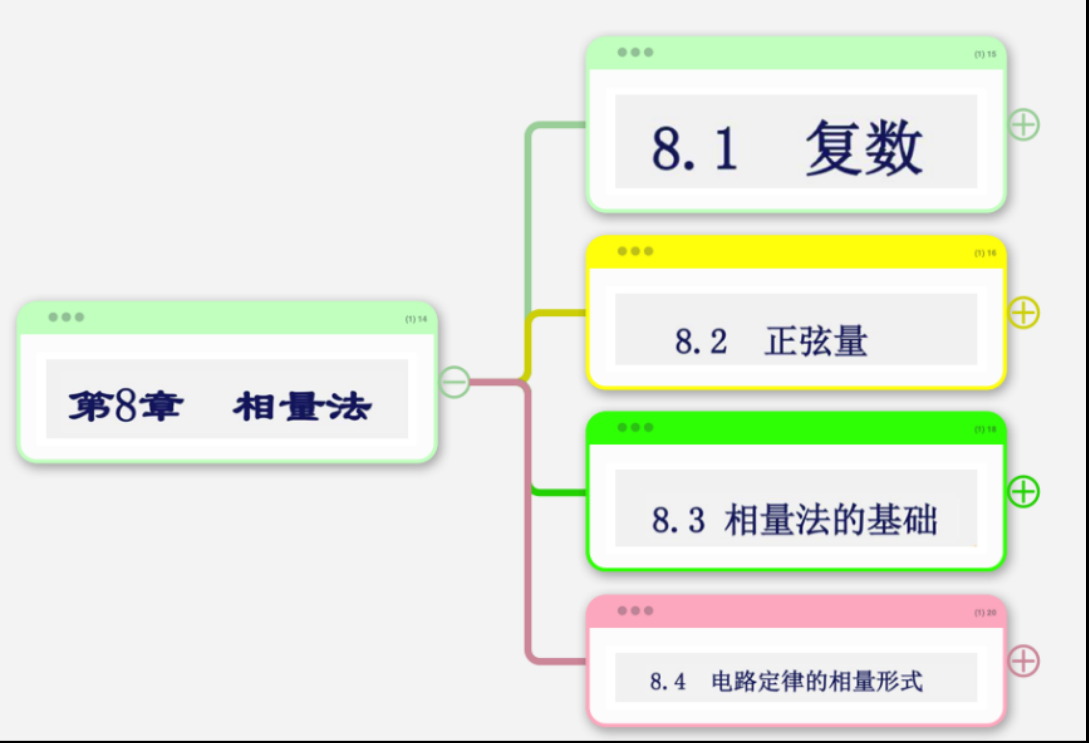## 复数

共四种形式，结合欧拉公式变换，其实就三种形式。他们分别是：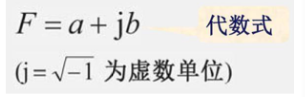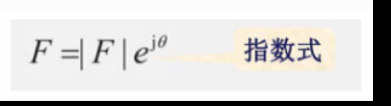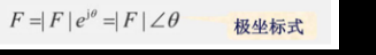结合欧拉公式，其实就是四种了，欧拉公式：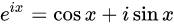可得：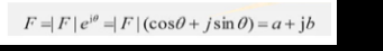欧拉公式的证明：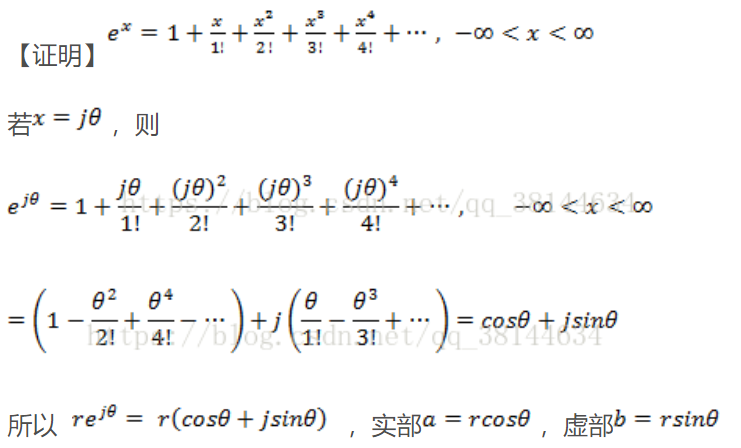幅值：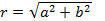相角：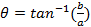## 复数的运算

复数相加（或相减）就是直接将它们的实部和虚部分别相加（或相减）进行：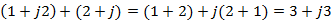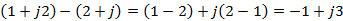在图形上，这个过程等同于两个矢量相加（或相减）的平行四边形定律（phasor）两个
复数相乘
可以将一个复数的每个部分乘以第二个复数的各个部分，然后将四个乘积相加，得到最终的值。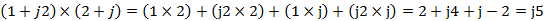在图形上，这个过程与矢量的内积并不相同，如果将每个复数看成复平面（实轴与虚轴组成）上的矢量，其相乘结果仍为一个复数，也就是说两个复数相乘结果为复平面上的一个矢量（复数），而矢量的内积则为一个实数。

不管是点乘还是叉乘，与两个复数相乘都不同
关于两个复数相乘还可以从其指数形式考虑，也就是著名的棣莫佛定理（De Moivre’s formula）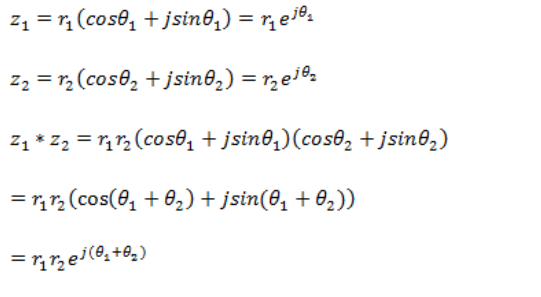棣莫夫定理也是我们利用相量法计算的一个重要依据，复指数形式相乘表现为幅值相乘，相角相加。我们如果用极坐标式来表示，也就是模相乘，角相加

## 相量法

相量法的基础在于这个关系式：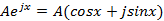由这个式子可以得到：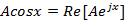其中Re[]是取实部的意思，对于正弦时变函数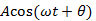可得：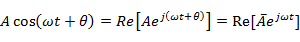其中，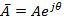称为对应于该正弦时变函数的相量，这是一个复数。也就是说对应于一个正弦时变函数的相量是一个复数，其模大小与余弦函数的幅度相同，其相位角等于 时刻余弦函数的相位。
相量引入的意义值得思考，相量作为一个复数引入正交时变函数直观上看将余弦函数的初相与其幅值合并，一定程度简化计算。
其简化计算的根本原因，还是欧拉公式与棣莫弗定律，由此二者得来四种复数表示形式，从而把复杂的运算转变成了简单的计算。
举一个例子，分析两个正弦时变函数（具有相同频率的）的加法: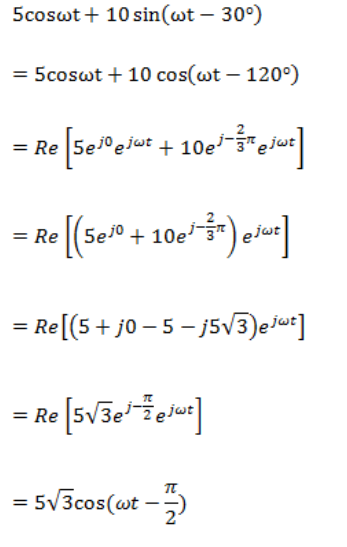展开全文• 动态向量法，研究动态向量 MMC 高压直流输电~~~~~~~~~~~~~~~~~~~~~~~~~~~~~~~~~~~
• 基于matlab对三维点云的法向量进行求取，并进行朝向统一。
• 动态向量法，研究动态向量 HVDC，非常好用~~~~~~~~~~~~~~~~~~~~~~~~~~~~~~~~~~~~~~~~~~~~~~~~~~~~~~~~~~~~~~~~~
• AHP层次分析计算权重、特征值、特征向量，第一作者刘兴，其他地方下载需要47个币 【 尚有疑问，欢迎沟通！！ 1.CSDN上有人说可用； 2.亲自试验，代码流畅，但个人感觉特征值、特征向量存疑； 3.用java求出的特征...
• 易语言源码易语言向量法计算文本相似度源码.rar
• 针对无任何预知信息下的散乱点云数据配准问题，提出了一种基于点云法向量信息的自动配准算法。根据点云局部法向量的变化提取特征点，通过比较特征点的直方图特征向量获得初始匹配点对；使用随机抽样一致性（RANSAC）...
• 1、运用k-近邻点估计点云法向量； 2、同时运用matlab自带的3D-pointcloud处理函数拟合点云法向量
• python open3d 计算法向量

法向量通常是针对于平面而言的，即垂直于平面的向量。因此，对于点云来说，需要先拟合出一个平面，然后才能求出相应的法向量。对于一个点，可以用周围邻近的点来拟合平面。

# 1 Open3d法向量计算方法

这里将采用Open3d来计算法向量，其对应的函数为estimate_normals。该函数的一个参数是搜索是定义平面的搜索参数。

如上文所述，计算法向量需要拟合出一个平面，这个平面有临近的点来决定。Open3d中提供三种方法来确定这些邻近的点。所有计算的法向量模长为1

o3d.geometry.KDTreeSearchParamKNN(knn=20)# 计算近邻的20个点
o3d.geometry.KDTreeSearchParamHybrid(radius=0.01, max_nn=20)#同时考虑搜索半径和近邻点个数

# 2 python open3d 计算法向量

点云数据下载地址：ply格式点云样例文件-深度学习文档类资源-CSDN下载

# -*- coding: utf-8 -*-
"""
Created on Sat Apr 16 08:51:41 2022

@author: https://blog.csdn.net/suiyingy
"""

import numpy as np
import open3d as o3d

if __name__ == '__main__':
file_path = 'bun000.ply'

pcd = o3d.geometry.PointCloud()
pcd.points = ply.vertices

max_nn = 30  # 邻域内用于估算法线的最大点数

normals = np.array(pcd.normals)#法向量结果，Nx3

#验证法向量模长为1
res = normals * normals
res = np.sum(res, axis=1)
print(res)

#法向量可视化
o3d.visualization.draw_geometries([pcd], window_name="法线估计",
point_show_normal=True,
width=800,  # 窗口宽度
height=600)  # 窗口高度



# 3 可视化结果

炸毛的兔子。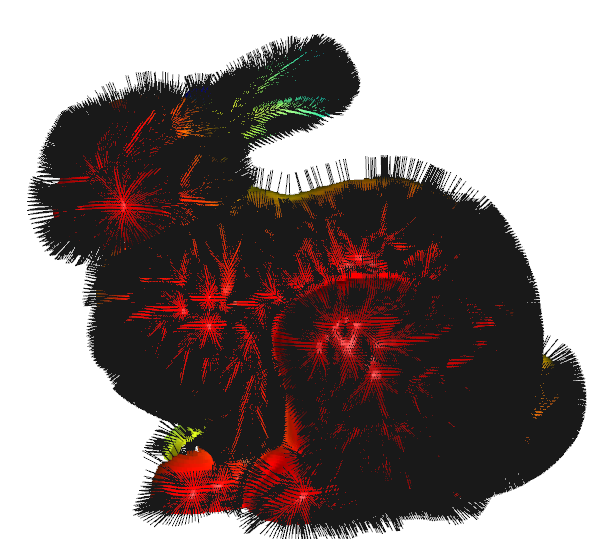# 4 Open3d注意事项

点云格式必须设置为pcd格式才能进行法向量计算。ply格式不支持法向量计算，需要按照上述代码中的方式转换为pcd之后才可以计算法向量。pcd文件读取后是可以直接进行法向量。展开全文点云 open3d python
• 易语言向量法计算文本相似度源码,向量法计算文本相似度,Similar_Text,初始化,生成CRC32表,取CRC32,去重复,取文本地址,指针到短整数_无符号,清零,取数组地址
• 根据读取文献，自己写的一个基于法向量的点云数据精简的算法，写的比较简单，有注释也通俗易懂。可以更改REM7的取值(0),来调整精简比例。
• 利用Matlab计算三维点云中每个点的单位法向量与特征值。 通过计算当前点与其邻域点的协方差矩阵，进而求得法向量
• 为了修复STL文件中的法向量错误，首先将有法向量错误的三角面片所围成的轮廓分为单个环、相连环以及环中环三种类型；然后采用如下的步骤来修复错误：找到所有有法向量错误的三角面片的边界边，找出边界边形成的封闭...STL文件
• 一些点云文件包括PCD格式和PLY格式，还有文件打开的代码和点云法向量计算代码等，直接运行可用
• 本书详细介绍了深度和法向量的非线性优化具体方法及公式推导。
• 基于局部平面拟合求点云法向量，是我看到过的比较容易懂的，算法推导也写得很清楚点云处理
• 机构运动分析的圆向量法，通过圆向量求解简单连杆机构的运动分析问题
• 在线结构光测量中，为快速准确地提取点云的法向量，提出了一种外接圆方法。通过图像细化求得光条纹初值中心点集，然后判定相邻三点是否共线：若共线，该点初始法线垂直于该直线；否则，这三点可以确定一个外接圆，...
• 对于三维点云的每一个点利用邻域点拟合最小二乘平面并求得法向量
•文档
• 基于支持向量机的分类，基于支持向量机的分类课件，基于支持向量机的分类PPT
• 了解表面的法向量可能很有用。 提供的函数能够采用 2.5D 无限制三角剖分（Delaunay 与否）来定义 3D 表面并计算每个单元格的法向量。 可以在中心单元或顶点处计算向量。 输入： “XYZ”是三角剖分的顶点坐标（nx3 ...matlab
• 本文简述了一种法向量变换矩阵的推导过程 使用矩阵对点进行空间变换是图形学中的常见操作,假设变换矩阵为 MMM ,我们需要变换切向量 TTT(由 点 P2P_2P2​ - 点 P1P_1P1​ 定义) 以及与其垂直的法向量 NNN .(TTT 和 ...

本文简述了一种法向量变换矩阵的推导过程

使用矩阵对点进行空间变换是图形学中的常见操作,假设变换矩阵为 M M ,我们需要变换切向量 T T (由 点 P 2 P_2 - 点 P 1 P_1 定义) 以及与其垂直的法向量 N N .( T T N N 皆为列向量)

假设 点 P 2 P_2 经过 M M 变换后为 P 2 ′ P_2&#x27; , 点 P 1 P_1 经过 M M 变换后为 P 1 ′ P_1&#x27; , T ′ T&#x27; 为经过变换后的切向量:

M ∗ P 2 = P 2 ′ M ∗ P 1 = P 1 ′ T ′ = P 2 ′ − P 1 ′ \begin{aligned} &amp; M * P_2 = P_2&#x27; \\ &amp; M * P_1 = P_1&#x27; \\ &amp; T&#x27; = P_2&#x27; - P_1&#x27; \end{aligned}

对于原切向量 T T ,我们希望找到一个矩阵 M ′ M&#x27; , 使得:

M ′ ∗ T = T ′ M&#x27; * T = T&#x27;

我们直接令 M ′ = M M&#x27; = M 来试一下:

M ′ ∗ T = M ∗ T = M ∗ ( P 2 − P 1 ) = M ∗ P 2 − M ∗ P 1 = P 2 ′ − P 1 ′ = T ′ \begin{aligned} M&#x27; * T = M * T &amp;= M * (P_2 - P_1) \\ &amp;= M * P_2 - M * P_1 \\ &amp;= P_2&#x27; - P_1&#x27; \\ &amp;= T&#x27; \end{aligned}

可见对于切向量 T T ,我们可以直接使用 M M 对其进行变换.

对于法向量 N N ,我们有(注意,第一个公式中的点号表示点积):

T ⋅ N = 0 &ThickSpace; ⟹ &ThickSpace; T T ∗ N = 0 \begin{aligned} &amp; T \cdot N = 0 \implies \\ &amp; T^T * N = 0 \end{aligned}

假设变换后的法向量为 N ′ N&#x27; , 我们希望仍然保持其与 T ′ T&#x27; ( T T 的变换后向量) 的垂直(注意,第一个公式中的点号表示点积):

T ′ ⋅ N ′ = 0 &ThickSpace; ⟹ &ThickSpace; T ′ T ∗ N ′ = 0 \begin{aligned} &amp; T&#x27; \cdot N&#x27; = 0 \implies \\ &amp; T&#x27;^T * N&#x27; = 0 \end{aligned}

假设用于变换法向量的矩阵为 G G , 则应有:

T ′ = M ∗ T N ′ = G ∗ N T ′ T ∗ N ′ = 0 ( M ∗ T ) T ∗ ( G ∗ N ) = 0 T T ∗ M T ∗ G ∗ N = 0 T T ∗ ( M T ∗ G ) ∗ N = 0 \begin{aligned} &amp; T&#x27; = M * T \\ &amp; N&#x27; = G * N \\ &amp; T&#x27;^T * N&#x27; = 0 \\ &amp; (M * T)^T * (G * N) = 0 \\ &amp; T^T * M^T * G * N = 0 \\ &amp; T^T * (M^T * G) * N = 0 \end{aligned}

由于我们知道:

T T ∗ N = 0 T^T * N = 0

所以我们只要令(注意,这只是一种可能的取值,并不是唯一取值,我们的目的也仅是需要获得一种可能的取值):

M T ∗ G = I M^T * G = I

便可以满足上面的等式 :

T T ∗ ( M T ∗ G ) ∗ N = T T ∗ I ∗ N = T T ∗ N = 0 \begin{aligned} &amp; T^T * (M^T * G) * N = \\ &amp; T^T * I * N = \\ &amp; T^T * N = 0 \end{aligned}

所以变换法向量,我们需要使用普通变化矩阵逆的转置(或者说转置的逆,对于可逆矩阵,其转置矩阵的逆矩阵等于其逆矩阵的转置矩阵)

G = ( M T ) − 1 = ( M − 1 ) T G = (M^T)^{-1} = (M^{-1})^T

如果变换矩阵 M M 是正交矩阵,则有(根据正交矩阵定义)

M − 1 = M T M^{-1} = M^T

于是法向量的变换矩阵变为:

G = ( M T ) − 1 = ( M − 1 ) − 1 = M G = (M^T)^{-1} = (M^{-1})^{-1} = M

此时我们就可以直接使用 M M 来变换法向量了.

#### 更多资料

展开全文变换矩阵 坐标变换
• ## matlab求法向量

千次阅读 2021-04-19 01:59:59
|dT/ds| dT/ds 投影方向单位向量,垂直于 T 平面 T 和 N 的单位法向量,即曲率的平面 曲线的扭率: |dB/ds| 重力常数 力学中力的标准符号 弹簧的弹簧常数 ......|dT/ds| dT/ds 投影方向单位向量,垂直于 T 平面 T 和 N ...
• 方向向量法向量的关系2020-07-17 09:39:11文/王君婷法向量是空间解析几何的一个概念，垂直于平面的直线所表示的向量为该平面的法向量。由于空间内有无数个直线垂直于已知平面，因此一个平面都存在无数个法向量...
• 求解点云向：（基于局部平面拟合点云的法向量） 点云法向量求解需要其邻域内点支持，而邻域的大小一般由邻域半径值或临近点个数来表示。现实中需要根据点分辨率、物体细节精细程度和用途等因素来取值。过大的邻域......